Select Page

# MCQ in English Matrices Solutions for CBSE Maths 12 Science

MCQ in English Matrices Solutions for CBSE Maths 12 Science to enable students to get Solutions in a narrative video format for the specific question.

Expert Teacher provides MCQ Matrices Solutions for CBSE Maths 12 Science through Video Solutions in English language. This video solution will be useful for students to understand how to write an answer in exam in order to score more marks. This teacher uses a narrative style for a question from Matrices not only to explain the proper method of answering question, but deriving right answer too.

Please find the question below and view the Solution in a narrative video format.

Question:

Solution Video in English:

You can select video Solutions from other languages also. Please check Solutions in ( Hindi )

## Similar Questions from CBSE, 12th Science, Maths, Matrices

Question 1 : If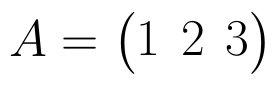, write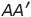, where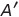is the transpose of A? (View Answer Video)

Question 2 :  Find the value of z, if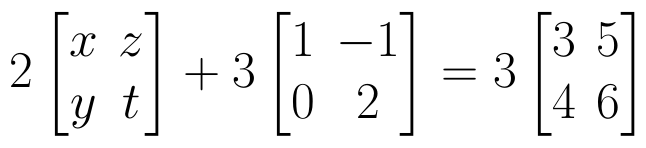(View Answer Video)

Question 3 : Let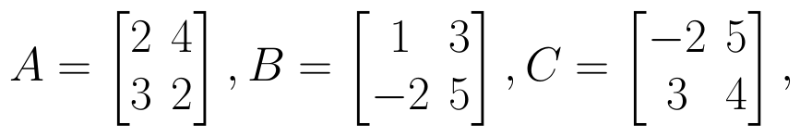Find 3A - C. (View Answer Video)

Question 4 : If,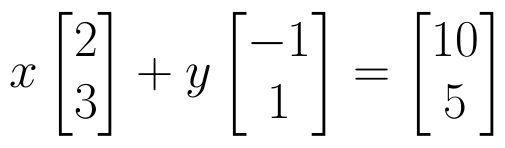find the value of y.  (View Answer Video)

Question 5 : Find the value of z,  from the equation: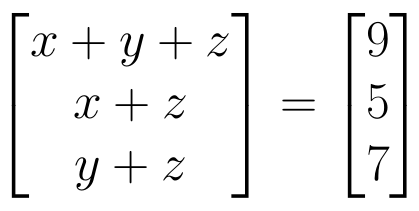. (View Answer Video)

### Determinant

Question 2 :  Evaluate the determinants: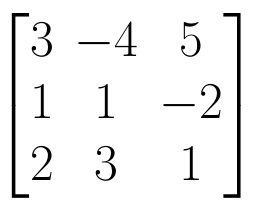. (View Answer Video)

Question 3 : Evaluate the determinant: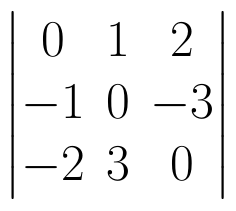. (View Answer Video)

Question 4 : If A =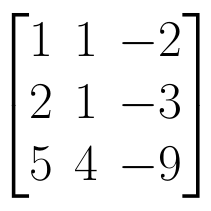, find | A |. (View Answer Video)

Question 5 : Evaluate the determinant: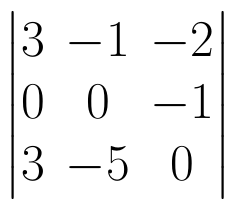. (View Answer Video)

### Integrals

Question 1 : Find the integral of the function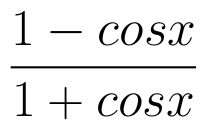. (View Answer Video)

Question 2 : Evaluate :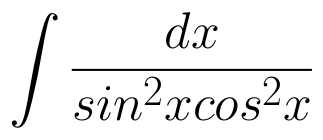(View Answer Video)

Question 3 : Evaluate :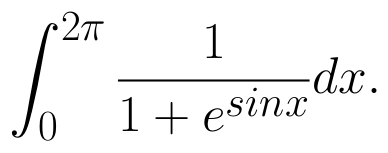(View Answer Video)

Question 4 : Evaluate :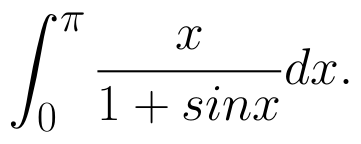(View Answer Video)

Question 5 : Evaluate :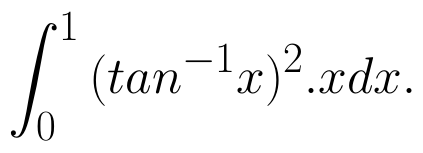(View Answer Video)

### Application of Integrals

Question 1 : Find the area bounded by the curve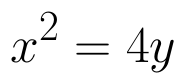and the line x = 4y - 2. (View Answer Video)

Question 2 : Find the area enclosed between the parabola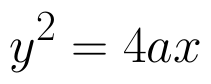and the line y = mx. (View Answer Video)

Question 3 : Using integration, find the area bounded by the tangent to the curve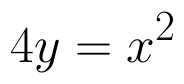at the point (2, 1) and the lines whose equations are x = 2y and x = 3y - 3. (View Answer Video)

Question 4 : Find the area enclosed by the parabola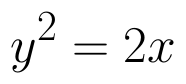and the line x - y = 4. (View Answer Video)

Question 5 : Find the area of the smaller region bounded by the ellipse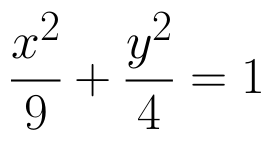and the line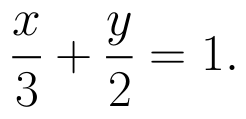(View Answer Video)